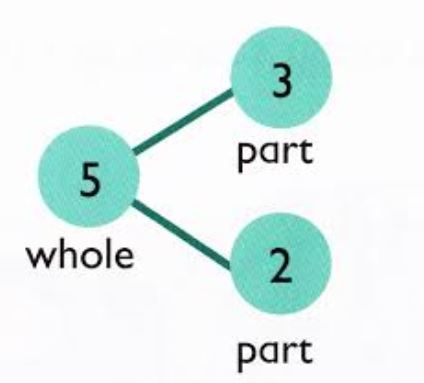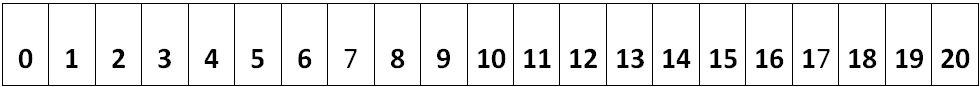1st Module 1--Sums and Differences to 10

Number bonds are  mental pictures of the relationships between a number and the parts that combine to make it.5 Group Cards represent numbers with dots. The top row fills to 5, then the bottom row to 10.This card shows 6 as 5 and 1 more.

equations:
3 + 2 = 5
2 + 3 = 5                                                    Equivalent equations:
5 = 3 + 2                                                    3 + 2 = 4 + 1
5 = 2 + 3                                                    5 + 0 = 3 + 2

3 + ? = 5
? + 2 = 5

Word Problems

Fluency in number bonds, addition and subtraction.

Should we add or subtract? Which is quicker?
10 - 9 = ? or 9 + ? = 10 whole - part = part or part + part = whole

Using the Number path to add and subtract (hop on to add, hop back to subtract)